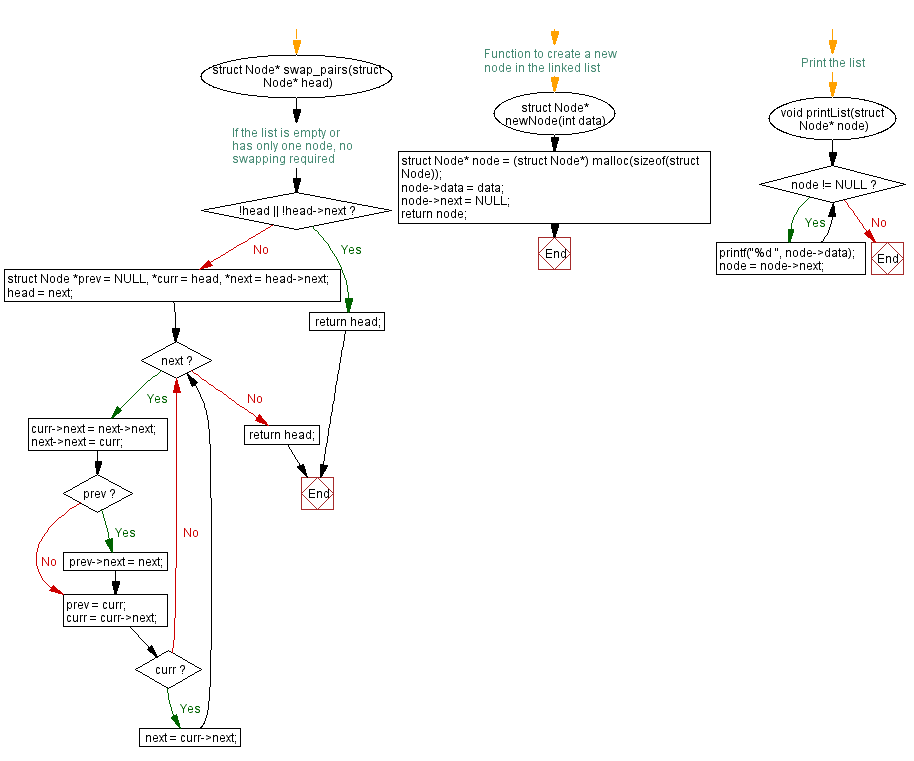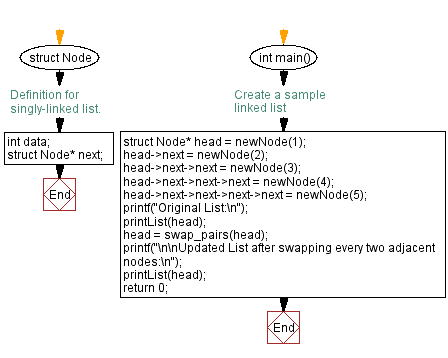﻿ C Program: Swap every two adjacent nodes of a singly linked list

# C Exercises: Swap every two adjacent nodes of a singly linked list

## C Singly Linked List : Exercise-40 with Solution

Write a C program to swap every two adjacent nodes of a given singly linked list.

Sample Solution:

C Code:

``````#include<stdio.h>
#include <stdlib.h>

struct Node {
int data;
struct Node* next;
};

struct Node* swap_pairs(struct Node* head) {
// If the list is empty or has only one node, no swapping required

while (next) {
curr->next = next->next;
next->next = curr;
if (prev) prev->next = next;
prev = curr;
curr = curr->next;
if (curr) next = curr->next;
}

}

// Function to create a new node in the linked list
struct Node* newNode(int data) {
struct Node* node = (struct Node*) malloc(sizeof(struct Node));
node->data = data;
node->next = NULL;
return node;
}

// Print the list
void printList(struct Node* node) {
while (node != NULL) {
printf("%d ", node->data);
node = node->next;
}
}

int main() {
// Create a sample linked list
printf("Original List:\n");
printf("\n\nUpdated List after swapping every two adjacent nodes:\n");
return 0;
}
```
```

Sample Output:

```Original List:
1 2 3 4 5

Updated List after swapping every two adjacent nodes:
2 1 4 3 5
```

Flowchart :C Programming Code Editor:

What is the difficulty level of this exercise?

﻿